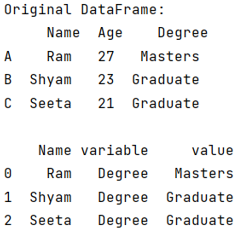# How to use melt function in pandas?

Given a Pandas DataFrame, we have to learn to use melt function.
Submitted by Pranit Sharma, on July 11, 2022

Pandas is a special tool that allows us to perform complex manipulations of data effectively and efficiently. Inside pandas, we mostly deal with a dataset in the form of DataFrame. DataFrames are 2-dimensional data structures in pandas. DataFrames consist of rows, columns, and data.

Many times, for a better understanding of datasets or to analyze the data according to our compatibility, we need to reorder or reshape the given DataFrame according to index and column values. The pandas.melt() method helps us to achieve this task.

## Pandas.melt() Method

The pandas.melt() method is useful when we need to unpivot a DataFrame, or whenever we need one or more columns as id_vars.

Syntax:

```pandas.melt(
frame,
id_vars=None,
value_vars=None,
var_name=None,
value_name='value',
col_level=None,
ignore_index=True
)
```

Parameter(s):

• frame: It is the DataFrame that we need to unpivot
• id_vars: columns which we need to specify as identifier variables
• value_vars: columns we need to unpivot
• var_name: The name of the column in which we will be acting as a variable

To work with pandas, we need to import pandas package first, below is the syntax:

```import pandas as pd
```

Let us understand with the help of an example,

## Python code to use melt function in pandas

```# Importing pandas package
import pandas as pd

# Creating a dictionary
d = {
'Name': {'A': 'Ram', 'B': 'Shyam', 'C': 'Seeta'},
'Age': {'A': 27, 'B': 23, 'C': 21},
}

# Creating a DataFrame
df = pd.DataFrame(d)

# Display original DataFrame
print("Original DataFrame:\n",df,"\n")

# melting DataFrame
print(pd.melt(df, id_vars =['Name'], value_vars =['Degree']))
```

Output:Top MCQs